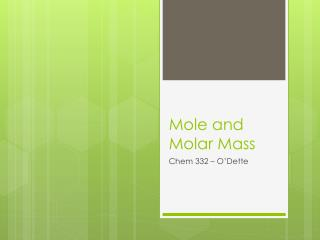DownloadDownload PresentationMole and Molar Mass

# Mole and Molar Mass

Télécharger la présentation## Mole and Molar Mass

- - - - - - - - - - - - - - - - - - - - - - - - - - - E N D - - - - - - - - - - - - - - - - - - - - - - - - - - -
##### Presentation Transcript

1. Mole and Molar Mass Chem 332 – O’Dette

2. Mole • A counting unit • 6.022x1023 particles in 1 mole • Aka Avogadro’s number, AN

3. Particles can be: • Atoms (elements) • 1 mole of carbon = 6.022x1023 atoms of C • Molecules (covalent compounds) • 1 mole of H2O = 6.022x1023 molecules of H2O • Formula Units (ionic compounds) • 1 mole NaCl = 6.022x1023 form. units NaCl = 6.022x1023 Na+ ions = 6.022x1023Cl- ions

4. Avogadro’s Number can be used as a conversion factor!!! 1 mole 6.022x1023particles OR 1 mole 6.022x1023 particles

5. Molar Mass (MM) • The mass of 1 mole of a compound or element • used as a conversion factor • equal to average atomic mass (from P.T.) • UNITS = grams mole

6. Ex: Find the MM of S • Look at the periodic table 32.066 g/mole 32.07 g/mole of S • Round to the hundredths place • Always label with the element/compound name

7. Ex: Find the MM of calcium nitrate • Ca(NO3)2 Element # of atomsMM = 40.08 =28.02 = +96.00 164.10 g Ca(NO3)2 mole Ca 1 x 40.08 14.01 N 2 x 6 O 16.00 x